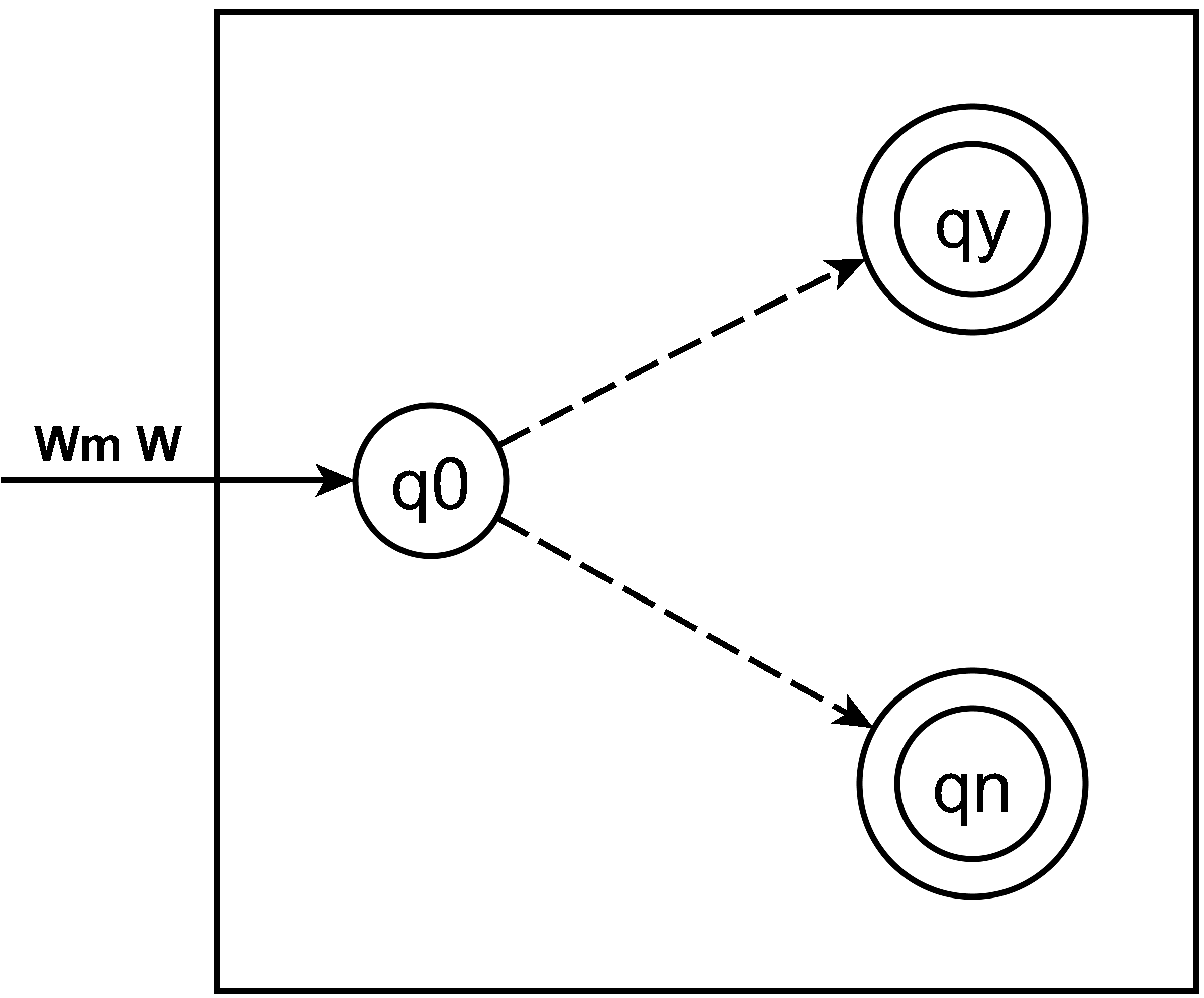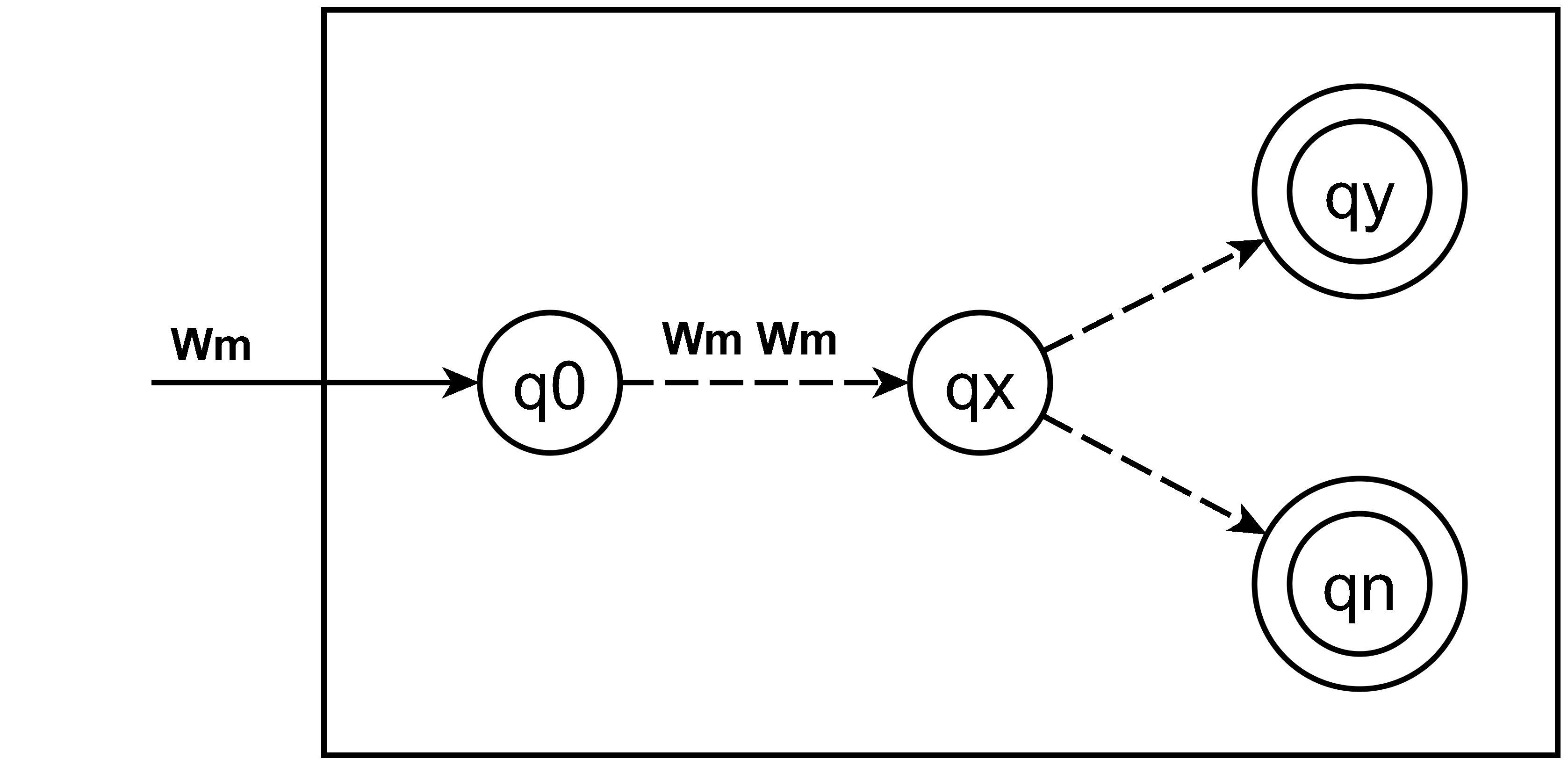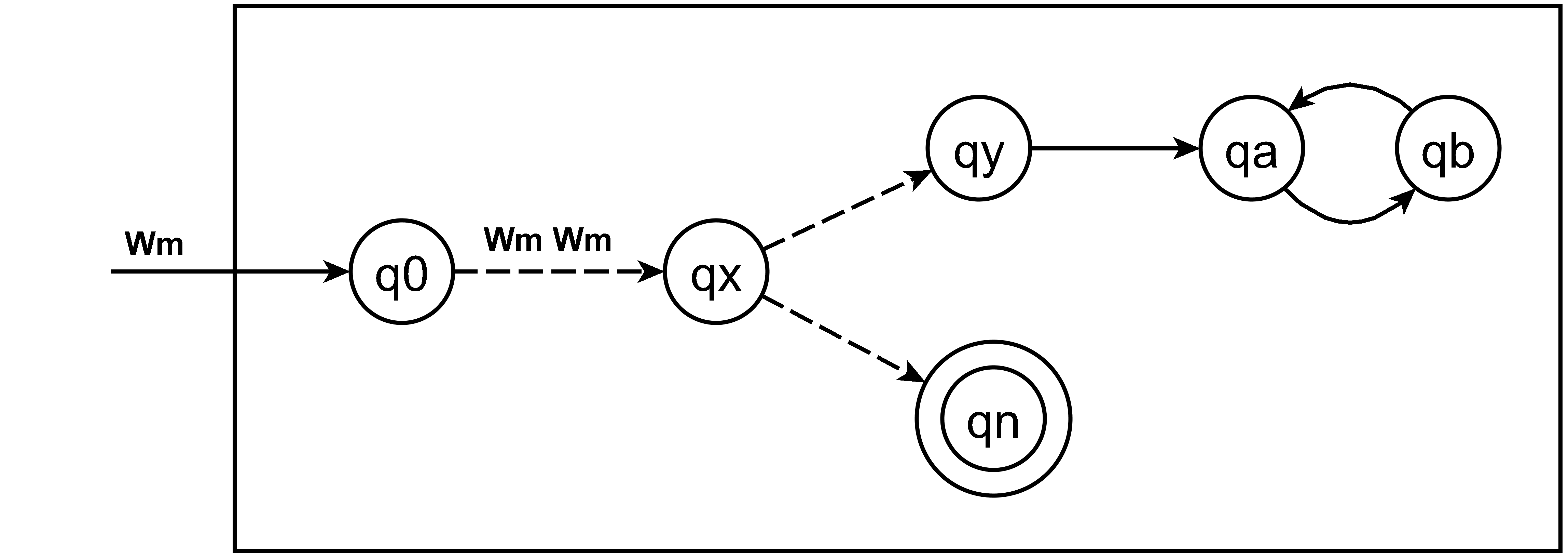# Refutation of Halting Problem Proof

Decidability of the Halting Problem as a David Hilbert formalism
∃a ∈ Turing_Machines_Descriptions
∀b ∈ Finite_Strings  // some of these specify Turing Machine Descriptions
∀c ∈ Finite_Strings
Halts(a, b, c) ∨ ~Halts(a, b, c)

Halt Decider definition
A Halt Decider (HD) determines whether or not a finite string represents a correct Turing Machine Description that would halt on its input. It does this by performing a mathematical proof on a pair of finite strings as a David Hilbert formalism in the language of Turing Machine Descriptions.

The syntactic logical consequence of this proof is two finite strings:
(1) “Y”  input pair (b,c) is a correct TMD that would halt on its input.
(2) “N” indicating the else branch of (1).

If the first element of the input finite string pair is a correct TMD then this proof must necessarily proceed from the specified TMD start state through all of the state transitions of this TMD to the halting or non halting behavior of this TMD.

The proof would essentially be a hypothetical execution trace** of the state transition sequences of the input TMD. It cannot be an actual execution trace or the halt decider could have non-halting behavior.
** Like step-by-step mode in a debugger.

We begin our analysis by constructing a hypothetical halt decider: H.

Figure 12.1 Turing Machine HThe dashed lines proceeding from state (q0) are represented in the text definition as the asterisk ⊢* wildcard character. These conventions are used to encode unspecified state transition sequences.

Definition of Turing Machine H (state transition sequence)
H.q0 Wm W ⊢* H.qy  // Wm W corresponds to (b,c) shown above
H.q0 Wm W ⊢* H.qn  // Wm W corresponds to (b,c) shown above

The diagram and the state transition sequence indicate that H begins at its own start state H.q0 and is applied to finite string pair (Wm, W).

H.qy is the final state of H indicating that Wm is a correct TM that would halt on input W.  Syntactic logical consequence : “Y” (shown above).

H.qn is the final state of H indicating that Wm is not a correct TM that would halt on input W.  Syntactic logical consequence: “N” (shown above).

We create Turing Machine Ĥx by making the following changes to H:
(1) Ĥx copies its input Wm a its Ĥx.q0 state and transitions to its Ĥx.qx state.

(2) Ĥx would begin to evaluate Wm Wm at its Ĥx.qx state in exactly the same way that H would begin to evaluate its Wm W input at its H.q0 state.

Since Turing Machine Ĥx is created by adapting H, it would have exactly the same behavior at its Ĥx.qx state as H would have at its H.q0 state.

Figure 12.4 Turing Machine ĤxDefinition of Turing Machine Ĥx (state transition sequence)
Ĥx.q0 Wm ⊢* Ĥx.qx Wm Wm ⊢* Ĥx.qy   // NO LOOP
Ĥx.q0 Wm ⊢* Ĥx.qx Wm Wm ⊢* Ĥx.qn   // NO LOOP HERE EITHER

If Turing Machine H is applied to Turing Machine description [Ĥx] [Ĥx]
would it transition to H.y or H.n ?

We append a “2” to the input to [Ĥx] for clarity [Ĥx2] is a copy of [Ĥx].

To answer this question we perform an execution trace on H
(1) H begins at its start state H.q0.
(2) H begins to evaluate what [Ĥx] would do on its input [Ĥx2].

Hypothetical Execution trace of what [Ĥ] would do if it was executed
(3) [Ĥx] would begin at its start state [Ĥx].q0
(4) [Ĥx] would make a copy of its input [Ĥx2], we will call this [Ĥx3].
(5) [Ĥx] would transition to its state [Ĥx].qx.
(6) [Ĥx] would begin to evaluate what [Ĥx2] would do on its input [Ĥx3].
Can you see the infinite recursion?

H [Ĥx] [Ĥx] specifies an infinitely recursive evaluation sequence.
Every HP proof by contradiction depends this same infinite recursion.

Because of this every TMD in the infinitely recursive sequence is defined in terms of H each would reject the whole sequence as semantically incorrect before even beginning any halting evaluation.

Therefore H would correctly transition to its H.qn state rejecting the infinitely recursive evaluation sequence specified by [Ĥx] [Ĥx] as the semantic error of Pathological self-reference(Olcott 2004).

Figure 12.3 Turing Machine ĤDefinition of Turing Machine Ĥ (state transition sequence)
Ĥ.q0 Wm ⊢* Ĥ.q Wm Wm ⊢* Ĥ.qy ∞
Ĥ.q0 Wm ⊢* Ĥ.q Wm Wm ⊢* Ĥ.qn

Copyright 2004, 2015, 2016, 2017, 2018 Pete Olcott